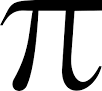# Radiometric conversion of sentinel 2 images

Hello all,

Just noticed this forum.

This has been working for me when converting my L1C rTOA to LTOA (top of atmosphere radiance).

LTOA = (rTOA * ESUN * cos(SunZe_grid)) / (pi * (d^2))

where the earth sun distance, d, at any time, in theory, can be calculated by just knowing the Julian Day the image was taken.

To find the earth sun distance…

First calculate the Julian day:

A = round(year ./ 100)

B = 2 - A + round(A / 4)

JD = round(365.25 * (year + 4716)) + round(30.6001 * (month + 1)) + day + (UT / 24.0) + B - 1524.5

(round just means the output should be in integer format)

Now find the earth sun distance!

D = JD - 2451545.0;
g = 357.529 + 0.98560028 .* D;
d = 1.00014 - 0.01671 .* cosd(g) - 0.00014 .* cosd(2 .* g);

Plug d into the first equation I provided above. Hope this helps.

Ben

What is ‘PI’ in the equation?

Hello,
I think its a count 3.14159…Correct me if I am in mistake

Regards

Thank you very much kiryl23

Hi All,
I am bit confused on the radiometric convertion. Is this on for reflectance value? What is the different with sen2cor approach?
Thanks

Hi Ben,
For this band math, should it be done before using the sen2cor or after?
In my research, I will using S2 and Landsat-8 for data fusion.
Regards

A lot has changed since I posted that answer. Maybe I can make your life easier. What reflectance product are you trying to achieve and maybe I can answer your question more thoroughly?

Ben

Not sure if you got my email. Anyway, Sentinel-2A images are in JP2000 format. When importing in a GIS or matrix laboratory, divide each band by the scaling factor (10,000) to retrieve TOA reflectance (rTOA as I have posted in the formula above). Use rTOA to retrieve TOA Radiance in the equation above. Hope this helps. Sorry it took me a while to reply.

Ben

Hi Ben,
Thank you for the information. Actually, I am bit confused on the radiometric convertion and sen2cor. Since I need the reflectance value for the fusion between S2 and L8. I wonder which is more practical to be used ( radiometric convertion and sen2cor) and whether it is the same process or can be integrated.
For example L8, I have already process up to atmospheric correction and convert it to reflectance value using band math. Is it the same process for S2?
Regards

sen2cor is a plugin designed for transforming Sentinel-2 MSI data on L1C (Top-of-atmosphere (TOA) reflectance) to L2A (Bottom-of-atmosphere (BOA) reflectance). So, when you want to perform atmospheric correction to the Sentinel-2 data in sen2cor, you don’t have to transform it to TOA radiance first. So, basically, you just have to input the image file and let the plugin works.

A different steps is required when you want to perform atmospheric correction with another algorithm (e.g. FLAASH, which is built-in in commercial software such as ENVI). As the algorithm needs TOA radiance data as the input, you have to tranform the data first, which is from TOA reflectance to TOA radiance, using equation given by Igor above (also see Distance of Earth-Sun). Performing atmospheric correction (e.g. FLAASH) allows you to have Surface reflectance data as the output.

Hi Fifauzan,
Thank you for the explanation. I think I know what to do now.
Regards,

Hello @fifauzan ,

Please, can i do the atmospheric correction with the ENVI software with Flaash algorithm

Hi @ELHACHIMI ,
Sorry for the late reply. This paper might help: https://www.researchgate.net/publication/307959143_Comparison_of_Physically_and_Image_Based_Atmospheric_Correction_Methods_for_Sentinel-2_Satellite_Imagery?ev=prf_high

1 Like

Hi @fifauzan,
Thank you very much for your help, this is a very important job for me, and this work will help me a lot.

1 Like

Hello @safah_94 ,